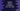# Python 3 program to check if a number is positive, negative or zero## Python 3 program to test if a number is positive or negative :

In this tutorial, we will learn how to test if a number is positive or negative. We will also check if the number is zero. This is a beginner-friendly python tutorial. With this example, you will learn how to read a user input, how to put your code in a different method to organize it, and how to use an_ if, else-if, else _condition. The program will take the number as input from the user, it will check if it is zero, greater than zero or less than zero and print out the result to the user. You can also store the number in a variable and check its value. But in this program, we are reading the number as an input from the user.

## Algorithm :

The algorithm of the program is like below :

1. Take the number as an input from the user. You can create one separate variable to hold the number or you can directly test the number. In this example, we are using one separate variable to hold it.
2. Check the number using one_ if-elseif-else_ condition. This condition will compare the number two times. The first one will check if it is equal to zero or not, the second one will check if it is greater than zero or not.

If both of these conditions fail, we will print that the number is less than zero or it is a negative number.

### Example Program :

``````def check_number(n):
if n == 0:
print ("Zero")
elif n > 0:
print (n,"is greater than zero")
else :
print (n,"is less than zero")

user_no = int(input("Enter a number : "))

check_number(user_no)``````